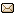Digital 논리게이트

## Digital 논리게이트 ¶

• 작성자
조재혁(minzkn@minzkn.com)

• 고친과정
2008년 어느날 : 처음씀

### OR의 교환법칙 ¶

```x OR y = y OR x
```

### OR의 결합법칙 ¶

```x OR y OR z = (x OR y) OR z = x OR (y OR z)
```

### AND : x AND y = F ¶

```0 AND 0 = 0
0 AND 1 = 0
1 AND 0 = 0
1 AND 1 = 1
```

### OR : x OR y = F ¶

```0 OR 0 = 0
0 OR 1 = 1
1 OR 0 = 1
1 OR 1 = 1
```

### Inverter : !x = F ¶

```!0 = 1
!1 = 0
```

```0 = 0
1 = 1
```

### NAND : x NAND y = !(x AND y) = F ¶

```0 NAND 0 = 1
0 NAND 1 = 1
1 NAND 0 = 1
1 NAND 1 = 0
```

### NOR : x NOR y = !(x OR y) = F ¶

```0 NOR 0 = 1
0 NOR 1 = 0
1 NOR 0 = 0
1 NOR 1 = 0
```

### XOR(Exclusive-OR) : x XOR y = (x AND !y) OR (!x AND y) = F ¶

```0 XOR 0 = 0
0 XOR 1 = 1
1 XOR 0 = 1
1 XOR 1 = 0
```

### Exclusive-NOR : x XNOR y = !(x XOR y) = (x AND y) OR (!x AND !y) = F ¶

```0 XNOR 0 = 1
0 XNOR 1 = 0
1 XNOR 0 = 0
1 XNOR 1 = 1
```